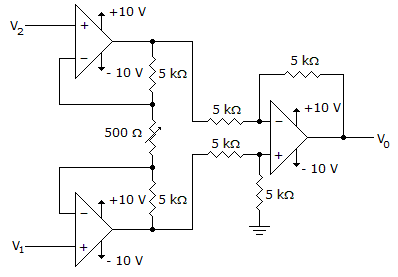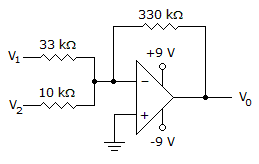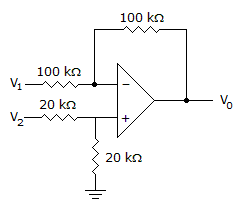# Electronic Devices - Op-Amp Applications

Exercise : Op-Amp Applications - General Questions
1.
Calculate the output voltage for this circuit when V1 = 2.5 V and V2 = 2.25 V.–5.25 V
2.5 V
2.25 V
5.25 V
Explanation:
No answer description is available. Let's discuss.

2.
Calculate the output voltage if V1 = –0.2 V and V2 = 0 V.0 V
–6.6 V
–4 V
2 V
Explanation:
No answer description is available. Let's discuss.

3.
A number of op-amp stages can be used to provide separate gains.
True
False
Explanation:
No answer description is available. Let's discuss.

4.
Determine the output voltage when V1 = –V2 = 1 V.0 V
–2 V
1 V
2 V
Explanation:
No answer description is available. Let's discuss.

5.
How many op-amps are required to implement this equation?

Vo = V1

2
3
4
1